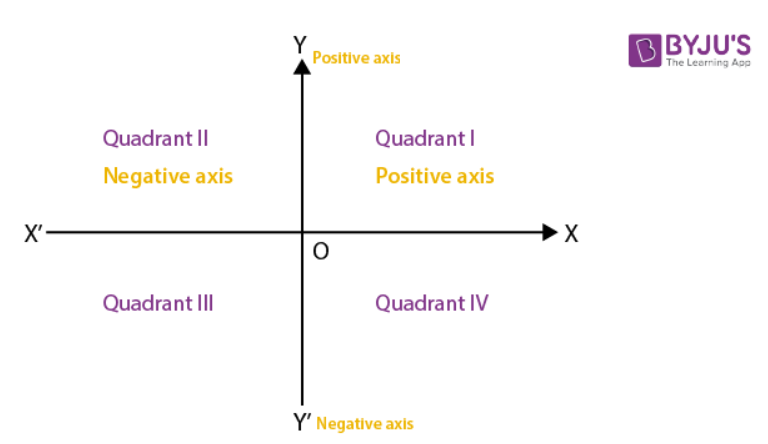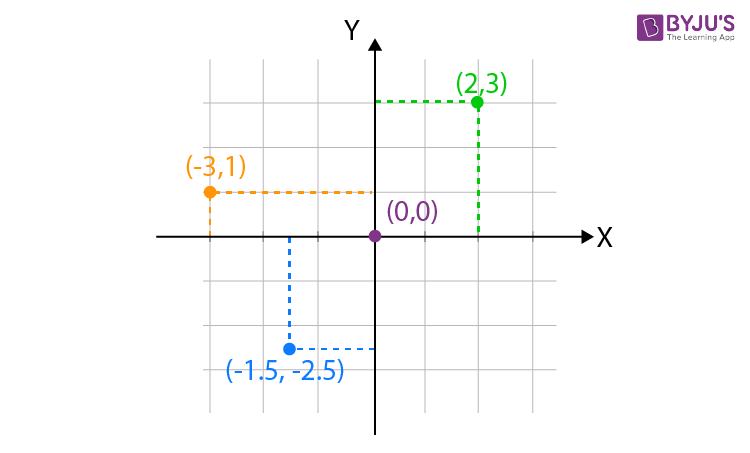# What is Cartesian Coordinate System?

Co-ordinate system is used to locate the position of a point in a plane using two perpendicular lines. Points are represented in the form of coordinates (x, y) in two-dimension with respect to x- and y- axes. In this article, we will learn about Cartesian Coordinate system.

To understand the need of coordinate system, let us consider an example, suppose Rina is a girl in your class and she sits on the 3rd column and 5th row. Then, this position can be represented as (3, 5).

Two axes – vertical axis and perpendicular axis are reference lines of a rectangular system from which distances are measured. They are obtained as follows:## Explanation:

Take two number lines XX’ and YY’. Place XX’ in horizontal and write the numbers on it as we write in the number line. Similarly, place YY’ in vertical and proceed writing numbers on it as we write in a number line. Combine both the lines in such a way that the two lines cross each other at their zeroes or origins. The horizontal line XX’ is called the x-axis and the vertical line YY’ is called the y-axis. The point where XX’ and YY’ cross is called the origin, and is denoted by O. Since the positive numbers lie on the directions OX and OY, OX and OY are called the positive directions of the x-axis and the y-axis respectively. Similarly, OX’ and OY’ are called the negative directions of the x- and y-axes respectively.

### Important Terms:

Moreover, the axes divide the plane into four parts and these four parts are called quadrants (one-fourth part). Thus, we have four quadrants numbered I, II, III and IV anticlockwise from OX.

Cartesian Plane:

A plane consists of axes and quadrants. Thus, we call the plane the Cartesian Plane, or the Coordinate Plane, or the x-y plane. The axes are called the coordinate axes.

Cartesian coordinate system for one dimensional:

The Cartesian coordinate system for one dimensional space consists of a line. We choose a point O, origin on the line, a unit of length and orientation for the line. The orientation chooses which of the two half lines determined by O is the positive, and which is negative. Each point P of the line can be specified by its distance from O, taken with a negative or positive sign .

Number line:

A line with a chosen Cartesian system is called a number line. Every real number has a unique location on the line. Every point on the number line can be interpreted as a number.

 Important Note: The above depicts a two-dimensional system. In case of a three-dimensional system, we have three mutually perpendicular axes, namely x, y and z. It can be generalised to create n coordinates for any point in n-dimensional Euclidean space.

### Abscissa and Ordinate

The x-coordinate of a point is its perpendicular distance from the y-axis measured along the x-axis and it is known as Abscissa.

The y-coordinate of a point is its perpendicular distance from the x-axis measured along the y-axis and it is known as Ordinate.

In writing the coordinates of a point in the coordinate plane, the x-coordinate comes first and then the y-coordinate. We place the coordinates in brackets as (x,y). The coordinates describe a point in the plane uniquely. It implies (3,1) ≠ (1,3) or in general (x,y) ≠ (y,x).

Consider an example point (5,6). Here abscissa = 5 and ordinate = 6.

Three dimensional geometry

Types Of Coordinate Systems

## Example Problems

Example 1: Locate below points on the cartesian coordinate system. Also, mention the quadrant points belong to.

(i) (2, 3)

(ii) (-3, 1)

(iii) (-1.5, -2.5)

(iv) (0,0)

Solution:Explanation:

(i) Point (2,3) marked by green dot in the graph. The horizontal distance is 2 units in positive x-direction and the vertical distance perpendicular from the reference horizontal axis is 3 units in positive y-direction and hence we denote the point as (2,3) in coordinate form and it belongs to quadrant I since both are positive. Similarly, we can extend this same idea to other points marked in the graph as well.

(ii) For the point marked in red colour, the horizontal distance is 3 units in negative x-direction and the vertical distance perpendicular from the reference horizontal axis is 1 unit in the positive y-axis and hence we denote the point as (-3,1) in coordinate form. It belongs to quadrant II as x-axis is negative and the y-axis is positive.

(iii) For the point marked in blue, the distance in horizontal and vertical units are respectively 1.5 and 2.5 both in negative directions. Thus, we have the point (-1.5.-2.5) in quadrant III.

(iv) Origin is the point of intersection of horizontal and vertical axes, which is (0, 0). It does not belong to any quadrant.

Example 2: If the vertices P, Q, R of a triangle PQR are rational points, which of the following points of the triangle PQR is (are) always rational point(s)
A) Centroid

B) Incentre

C) Circumcentre

D) Orthocentre (A rational point is a point both of whose coordinates are rational numbers)

Solution:

If $A=({{x}_{1}},\,\,{{y}_{1}}),\,\,B=({{x}_{2}},\,\,{{y}_{2}}),\,\,C=({{x}_{3}},\,\,{{y}_{3}}),$ where ${{x}_{1}},\,\,{{y}_{1}},$ etc., are rational numbers then

$\Sigma {{x}_{1}},\,\,\Sigma {{y}_{1}}$ are also rational. So, the coordinates of the centroid $\left( \frac{\Sigma {{x}_{1}}}{3},\,\,\frac{\Sigma {{y}_{1}}}{3} \right)$ will be rational.

As $AB=c=\sqrt{{{({{x}_{1}}-{{x}_{2}})}^{2}}+{{({{y}_{1}}-{{y}_{2}})}^{2}},}\,\,c$ may or may not be rational and it may be an irrational number of the form $\sqrt{p}.$

Hence, the coordinates of the incentre $\left( \frac{\Sigma a{{x}_{1}}}{\Sigma a},\,\,\frac{\Sigma a{{y}_{1}}}{\Sigma a} \right)$ may or may not be rational.

If $(\alpha ,\,\,\beta )$ be the circumcentre or orthocentre, a and b are found by solving two linear equations in $\alpha ,\,\,\beta$ with rational coefficients. So $\alpha ,\,\,\beta$ must be rational numbers.

If the vertices of PQR are rational points, then the centroid, the orthocentre and circumcentre will always be rational points.
Therefore, Options A), B) and C) are correct,

Example 3: Let A(2, -3) and B(-2, 1) be vertices of a triangle ABC. If the centroid of this triangle moves on the line 2x + 3y = 1, then the locus of the vertex C is the line _____.

Solution: Let the third vertex be (x1,y1) then centroid $(G)\equiv \left( \frac{{{x}_{1}}+2-2}{3},\,\frac{{{y}_{1}}-3+1}{3} \right)$ i.e., $G\,\left( \frac{{{x}_{1}}}{3},\,\frac{{{y}_{1}}-2}{3} \right)$

Given, centroid of triangle moves on the line

2x+3y =1

So 2(x1/3)+3(y1-2)/3 = 1

i.e.,  $2{{x}_{1}}+3{{y}_{1}}=9$

Locus of (x1,y1) is 2x+3y=9

Example 4: One of the vertices of a square is origin and adjacent sides of the square are coincident with positive axes. If side is 5 then which will not be its one of the vertex

(A) (0, 5)

(B) (5, 0)

(C) (- 5, -5)

(D) (0, 0)

Solution:

The square lies in first quadrant. So (-5, -5) will not be a vertex.

Hence option C is the answer.

Test Your Knowledge On What Is Cartesian Coordinate System!